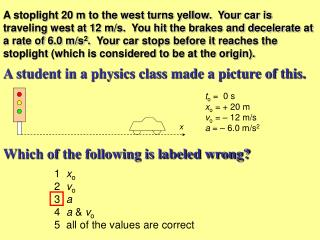DownloadDownload PresentationA student in a physics class made a picture of this.

# A student in a physics class made a picture of this.

Download Presentation## A student in a physics class made a picture of this.

- - - - - - - - - - - - - - - - - - - - - - - - - - - E N D - - - - - - - - - - - - - - - - - - - - - - - - - - -
##### Presentation Transcript

1. x A stoplight 20 m to the west turns yellow. Your car is traveling west at 12 m/s. You hit the brakes and decelerate at a rate of 6.0 m/s2. Your car stops before it reaches the stoplight (which is considered to be at the origin). A student in a physics class made a picture of this. to = 0 s xo = + 20 m vo = – 12 m/s a = – 6.0 m/s2 Which of the following is labeled wrong? 1 xo 2 vo 3 a 4 a & vo 5 all of the values are correct

2. A stoplight 20 m to the west turns yellow. Your car is traveling west at 12 m/s. You hit the brakes and decelerate at a rate of 6.0 m/s2. Your car stops before it reaches the stoplight (which is considered to be at the origin). x A student in a physics class made a picture of the situation but did not include the signs of the variables. to = 0 s xo = __ 20 m vo = __ 12 m/s a = __ 6.0 m/s2 What are the signs of the three missing variables? xovoa 1 + – – 2 + – + 3 + + – 4 + + + 5 – – +

3. vfinal vinitial a x t 1 2 3 v v v t t t v 4 The motion of a car is represented with the motion diagram below. Which graph best matches the motion diagram? • No graph represents the motion correctly

4. 3 1 2 x x x t t t 4 x t A stoplight 20 m to the west turns yellow. Your car is traveling west at 12 m/s. You hit the brakes and decelerate at a rate of 6.0 m/s2. Your car stops before it reaches the stoplight (which is considered to be at the origin). Which graph is the best representation of the car’s motion? • No graph represents the motion correctly

5. t v A bicycle is moving on the road. Its motion is described by the graph below. Below is a mathematical description of the same motion. What should the signs of vo and a be to be consistent with the above graphical description? voa 1 + – 2 + + 3 – + 4 – –

6. A hot air balloon is stationary in the sky as seen in the picture. A person throws a ball up in the air at 5 m/s. Which set of equations best matches this process? 1. Vf = (5.0 m/s) + (10 m/s2) t 2. Vf = ( - 5.0 m/s) + (10 m/s2) t 3. Vf = (5.0 m/s) + ( - 10 m/s2) t 4. Vf = ( - 5.0 m/s) + ( - 10 m/s2) t 5. Either 2 or 3 are correct

7. 2 3 x 1 x x t t t 4 x t The mathematical representation seen below is in a physics textbook. Which graph best represents this situation? • Graphs 3 & 4 • Graphs 2, 3 & 4 • No graph represents the motion correctly

8. Susy sees the mathematical representation below in her physics book. Which description best represents this situation? • This is a car that is moving to the right and is accelerating in the same direction. • A ball was thrown upwards in the air at a speed of 20 m/s. • A ball was thrown downwards at a height of 5 m at a speed of 20 m/s.

9. v x t t Alan sees the following three diagrams next to each other in his physics book. a t A B C Are these three diagrams consistent with each other? • Yes, all three diagrams are consistent. • No, Diagram A is not consistent with the other two. • No, Diagram B is not consistent with the other two. • No, Diagram C is not consistent with the other two. • None of the diagrams are consistent.

10. vinitial vfinal x + A car is moving along the road as seen in the motion diagram below. Which set of equations best matches this process? 1. Vf = (5.0 m/s) + (10 m/s2) t 2. Vf = ( - 5.0 m/s) + (10 m/s2) t 3. Vf = (5.0 m/s) + ( - 10 m/s2) t 4. Vf = ( - 5.0 m/s) + ( - 10 m/s2) t 5. Either 2 or 3 are correct